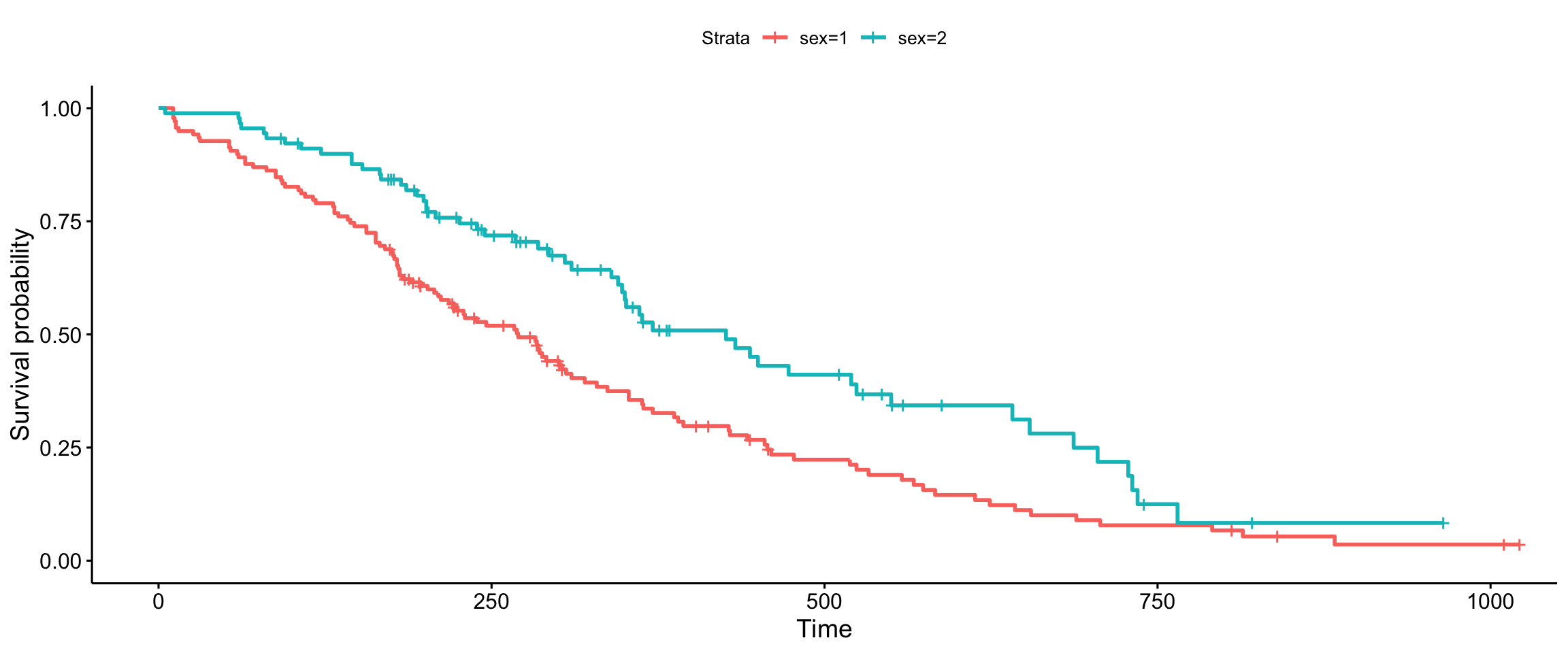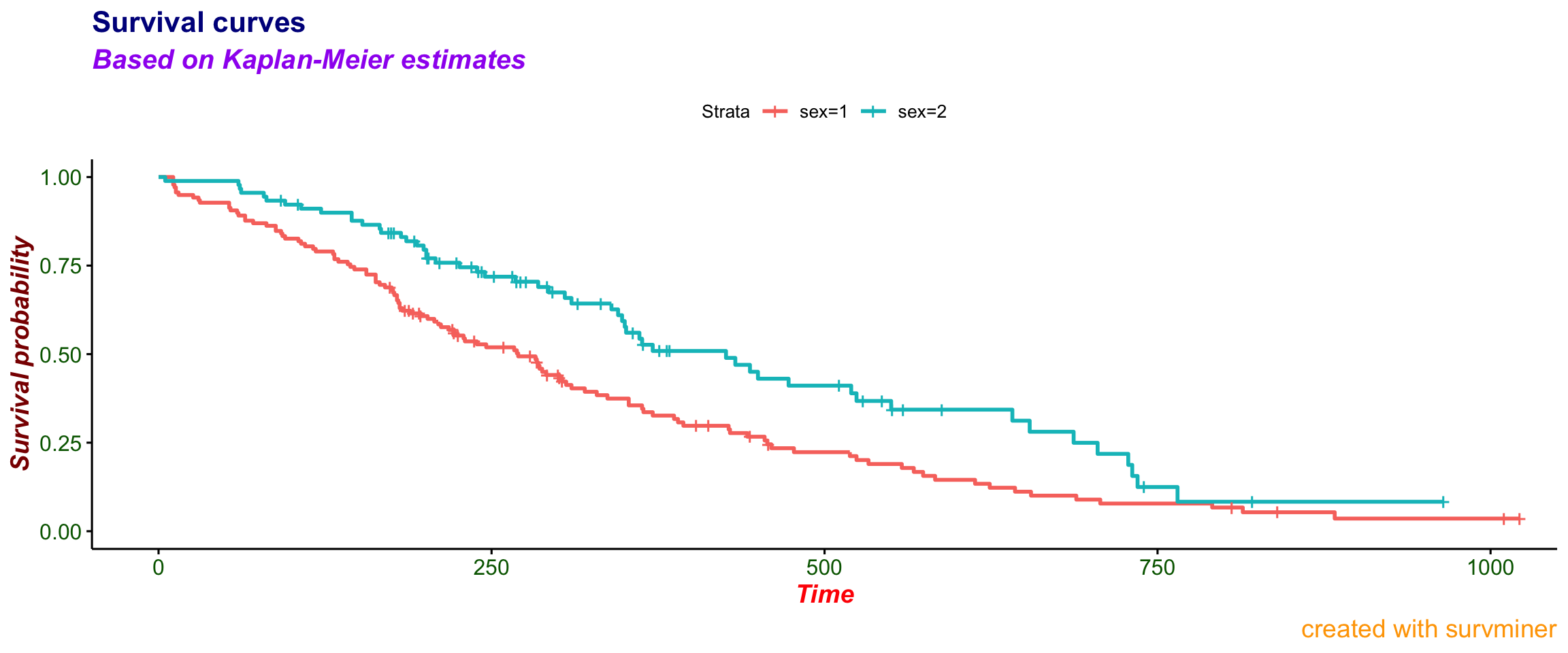``library("survminer")``

This vignette covers changes between versions 0.2.4 and 0.2.5 for additional texts and fonts customization enabled for subtitles and captions.

# Survival plot

## Basic

Compare the basic plot

``````library("survival")
fit<- survfit(Surv(time, status) ~ sex, data = lung)

# Drawing survival curves
ggsurvplot(fit, data = lung)``````## Customized

with the plot where every possible text on a `plot` is specified

``````ggsurvplot(fit, data = lung,
title = "Survival curves", subtitle = "Based on Kaplan-Meier estimates",
caption = "created with survminer",
font.title = c(16, "bold", "darkblue"),
font.subtitle = c(15, "bold.italic", "purple"),
font.caption = c(14, "plain", "orange"),
font.x = c(14, "bold.italic", "red"),
font.y = c(14, "bold.italic", "darkred"),
font.tickslab = c(12, "plain", "darkgreen"))``````# Risk table

Now allow `risk.table` to be displayed.

## Basic

Please compare basic plot with a risk table

``ggsurvplot(fit, data = lung, risk.table = TRUE)``